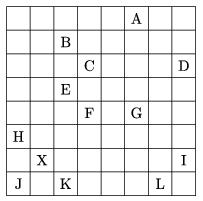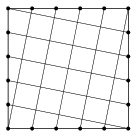Mathematical and Physical Journal
for High Schools
Issued by the MATFUND Foundation
 Already signed up? New to KöMaL?

# KöMaL Problems in Mathematics, October 2016

Show/hide problems of signs:## Problems with sign 'K'

Deadline expired on November 10, 2016.

K. 511. A merchant regularly buys apricot jam from a canning factory. One day, he bought a certain quantity, and paid a total of $\displaystyle 180\,000$ forints (HUF, Hungarian currency) for it. In the next month, he saw that the price of apricot jam was significantly reduced: they were selling 5 jars of jam for the price of 3 jars. The merchant was so happy that he immediately bought four times the quantity he had bought in the previous month. How much did he pay altogether for the jam with reduced price?

(6 pont)

solution (in Hungarian), statistics

K. 512. Patrick, Peter and Paul are triplets. One day, they were walking with their mom, and they saw a chewing gum vending machine. It contained a mixture of gums of three different colours (red, yellow and green). 100 forints (HUF, Hungarian currency) need to be inserted in the machine to get a chewing gum. The triplets insisted on getting gums of the same colour. Their mom kept throwing coins in the machine until she was able to fulfil the children's wish. They walked the same route on four consecutive days, and bought chewing gum in the same way every day. What is the maximum amount of money the mom may have spent on chewing gum, if she did all she could to economize?

(6 pont)

solution (in Hungarian), statistics

K. 513. The diagram shows a chessboard with chessmen on it. There is a bishop on the field marked X, and there are pawns on the other fields marked with letters. One by one, eliminate the pawns with the bishop, so that it captures a pawn in every move. Find a possible order by specifying the corresponding letters of the pawns.(The task can be found on www.sakkpalanta.hu, along with several other puzzles of this kind.)

(6 pont)

solution (in Hungarian), statistics

K. 514. A certain two-digit number is equal to seven times the sum of its digits. By what factor is the reversed two-digit number greater than the sum of the digits?

(6 pont)

solution (in Hungarian), statistics

K. 515. Each side of a regular hexagon is extended to the double of its length in the same direction around the clock. The points obtained are connected to form a new hexagon. By what factor is the area of the new hexagon greater than the area of the original hexagon?

(6 pont)

solution (in Hungarian), statistics

K. 516. Consider the sets $\displaystyle A = \{a, 2a+1, a^{2}+1\}$, $\displaystyle B =\{b+3, 10, b-1\}$. Find appropriate positive integers $\displaystyle a$ and $\displaystyle b$ such that the two sets have

$\displaystyle a)$ no element in common;

$\displaystyle b)$ exactly 1 element in common;

$\displaystyle c)$ exactly 2 elements in common;

$\displaystyle d)$ the same elements.

(6 pont)

solution (in Hungarian), statistics## Problems with sign 'C'

Deadline expired on November 10, 2016.

C. 1371. Each side of a unit square is divided into $\displaystyle n$ equal parts, and the division points of opposite sides are connected in a shifted manner, as shown in the diagram (representing the case of $\displaystyle n=5$). Prove that it is possible to reassemble these pieces to get $\displaystyle n^2+1$ smaller congruent squares.(5 pont)

solution (in Hungarian), statistics

C. 1372. A regular octagon is formed by cutting off the corners of a square in the appropriate way. Which is greater: the percentage decrease of the perimeter of the square or the percentage decrease of its area?

(5 pont)

solution (in Hungarian), statistics

C. 1373. Let $\displaystyle a$ and $\displaystyle b$ denote positive integers. Given that three line segments of lengths $\displaystyle a$, $\displaystyle 1/a$, $\displaystyle b$ can form a triangle, and three line segments of lengths $\displaystyle a$, $\displaystyle b$, $\displaystyle 1/b$ can also form a triangle, prove that both triangles are isosceles.

(5 pont)

solution (in Hungarian), statistics

C. 1374. The sides of a kite are 6 cm and 8 cm long, and sides of different lengths enclose a right angle. Determine the distance between the centres of the inscribed circle and the circumscribed circle of the kite.

(5 pont)

solution (in Hungarian), statistics

C. 1375. How many two-digit numbers are there in base $\displaystyle n$ notation, whose digit sum is also a two-digit number (in base $\displaystyle n$)? State your answer in base $\displaystyle n$.

(5 pont)

solution (in Hungarian), statistics

C. 1376. The roots of the equation $\displaystyle x^2+px+q=0$ are non-zero integers. Show that $\displaystyle p^2+{(q-1)}^2$ is a composite number.

(5 pont)

solution (in Hungarian), statistics

C. 1377. An isosceles triangle of perimeter 20 cm is rotated about its base. What is the maximum possible volume of the double cone obtained?

(5 pont)

solution (in Hungarian), statistics## Problems with sign 'B'

Deadline expired on November 10, 2016.

B. 4813. Given a prime $\displaystyle p$, find the integer solutions of the equation

$\displaystyle \left|\frac{2}{x}-\frac{1}{y}\right|=\frac{1}{p}.$

(3 pont)

solution (in Hungarian), statistics

B. 4814. Let $\displaystyle P$ be a given point in the interior of a sphere. Consider the pairwise perpendicular planes $\displaystyle S_1$, $\displaystyle S_2$ and $\displaystyle S_3$ that intersect at $\displaystyle P$. Show that the sum of the areas of the circles cut out of the planes by the sphere is independent of the choice of the planes $\displaystyle S_1$, $\displaystyle S_2$ and $\displaystyle S_3$.

(Italian problem)

(4 pont)

solution (in Hungarian), statistics

B. 4815. The binary operation $\displaystyle \circ$ is defined on the set of integers. Given that

$\displaystyle a\circ(b+c)=b\circ a+ c\circ a.$

for all integers $\displaystyle a$, $\displaystyle b$ and $\displaystyle c$, show that there exists an integer $\displaystyle k$ such that $\displaystyle a\circ b= k\cdot a \cdot b$ for all $\displaystyle a$ and $\displaystyle b$.

(Italian problem)

(5 pont)

solution (in Hungarian), statistics

B. 4816. Let $\displaystyle O$ denote the midpoint of line segment $\displaystyle AB$, and let $\displaystyle C$ be the point on line segment $\displaystyle OB$ for which $\displaystyle \frac{AO}{OC}=\frac{OC}{CB}$. Point $\displaystyle D$ is one of the points where the perpendicular drawn at point $\displaystyle C$ to $\displaystyle AB$ intersects the Thales circle of $\displaystyle AB$. Points $\displaystyle E$ and $\displaystyle F$ are the two intersections of line $\displaystyle AD$ with the circle of radius $\displaystyle OC$ centred at $\displaystyle O$. Determine the size of the angle $\displaystyle EOF$.

Proposed by Sz. Miklós, Herceghalom

(3 pont)

solution (in Hungarian), statistics

B. 4817. Solve the following equation in the set of real numbers:

$\displaystyle x + y + z = xyz = 8,$

$\displaystyle \frac 1x- \frac 1y- \frac 1z = \frac 18.$

Proposed by B. Kovács, Szatmárnémeti

(4 pont)

solution (in Hungarian), statistics

B. 4818. The intersection of diagonals $\displaystyle AC$ and $\displaystyle BD$ of a cyclic quadrilateral $\displaystyle ABCD$ is $\displaystyle M$. The angle bisectors of the angles $\displaystyle \angle CAD$ and $\displaystyle \angle ACB$ intersect the circumscribed circle of the cyclic quadrilateral $\displaystyle ABCD$ at points $\displaystyle E$ and $\displaystyle F$, respectively. Prove that line $\displaystyle EF$ is perpendicular to the angle bisector of the angle $\displaystyle AMD$.

Proposed by B. Bíró, Eger

(5 pont)

solution (in Hungarian), statistics

B. 4819. Prove that if $\displaystyle 0<x<\frac{\pi}{2}$, then

$\displaystyle {(\tg x)}^{\sin x}+ {(\ctg x)}^{\cos x}\ge 2.$

For what values of $\displaystyle x$ does the equality hold?

(Kvant)

(5 pont)

solution (in Hungarian), statistics

B. 4820. Four lattice points in a lattice of regular triangles of unit side form the vertices of a parallelogram $\displaystyle \mathcal{P}$. The area of $\displaystyle \mathcal{P}$ is $\displaystyle \sqrt{3}$ units. What may be the sum of the lattice segments in the interior of $\displaystyle \mathcal{P}$?

(6 pont)

solution (in Hungarian), statistics

B. 4821. Does there exist an integer $\displaystyle a\ge 1$ such that $\displaystyle x^2+3$ and $\displaystyle {(x+a)}^2+3$ are relatively primes for all positive integers $\displaystyle x$?

Proposed by D. Kovács

(6 pont)

solution (in Hungarian), statistics## Problems with sign 'A'

Deadline expired on November 10, 2016.

A. 677. Find all pairs $\displaystyle (p,q)$ of (positive) prime numbers for which $\displaystyle p^3+q^3+1$ is divisible by $\displaystyle pq$.

(5 pont)

statistics

A. 678. The convex polyhedron $\displaystyle \mathcal{K}$ has five vertices, $\displaystyle A$, $\displaystyle B$, $\displaystyle C$, $\displaystyle D$ and $\displaystyle E$. The line segment $\displaystyle DE$ intersects the plane of the triangle $\displaystyle ABC$ in the interior of the triangle. Show that $\displaystyle \mathcal{K}$ has an inscribed sphere – with being tangent to all six faces – if and only if the inscribed spheres of the terahedra $\displaystyle ABCD$ and $\displaystyle ABCE$ are tangent to each other.

(5 pont)

statistics

A. 679. Let $\displaystyle n = 2^{128}$, $\displaystyle M = \{1, 2, 3, 4\}$, and let $\displaystyle M^n$ denote the set of sequences of length $\displaystyle n$ and consisting of elements of $\displaystyle M$. Decide whether there exist functions $\displaystyle f_1,\ldots,f_n$ and $\displaystyle g_1,\ldots,g_n\colon M^n\to M$ such that for every pair of sequences

$\displaystyle (x_1,\ldots,x_n),(y_1,\ldots,y_n)\in M^n,$

at least one of the following statements holds:

$\displaystyle \bullet$ $\displaystyle f_i(y_1,\ldots,y_n) = x_i$ for some index $\displaystyle i$ with $\displaystyle 1\le i\le n$;

$\displaystyle \bullet$ $\displaystyle g_j(x_1,\ldots,x_n) = y_j$ for some index $\displaystyle j$ with $\displaystyle 1\le j\le n$.

Proposed by: Gerhard Woeginger, Eindhoven

(5 pont)

solution, statistics